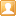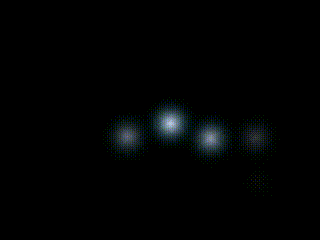##用户名 Email 自动登录 找回密码 密码 立即注册
 搜索

# [转载] Ren'Py引擎从入门到放弃(支线3·续)——简单粒子效果发表于 2019-4-25 19:05:54 | 显示全部楼层 |阅读模式

### 马上注册，结交更多好友，享用更多功能，让你轻松玩转社区。

×

Ren'Py引擎从入门到放弃(支线3·续)——简单粒子效果

class ParticleBurst(renpy.Displayable)定义表示从renpy.Displayable继承。后面的 __init__rendervisit 三连是ParticleBurst继承后覆盖(override)的3个成员函数。官方文档样例里还有个 event函数，是用来响应事件消息的，一般用在按钮之类的组件上。
[RenPy] 纯文本查看 复制代码
`def __init__(self, displayable, interval=(0.02, 0.04), speed=(0.15, 0.3), around=(config.screen_width/2, config.screen_height/2), angle=(0, 360), radius=(50, 75), particles=None, mouse_sparkle_mode=False, **kwargs)`

__init__ 函数有一堆入参，self 表示自身的指针，**kwargs 表示额外参数列表指针，这两个是固定的。其他几个参数的定义如下：

• displayable：Ren'Py中可以显示的任意内容(除了包含随意内容的可视组件或者可视组件容器。)
• interval: ：每次生成粒子的时间间隔，单位为秒。入参格式是一个二元的元组，元素值是浮点数。在两个数值之间生成一个随机数作为时间间隔。
• speed：粒子发射速度。也是二元的元组，两个数值之间生成一个随机数作为速度。
• angle：粒子发射角度。也是二元的元组，两个数值之间生成一个随机数。
• around：发射中心坐标，也是二元的元组，(x, y)形式的坐标值。所有粒子都会从这个点发射。
• particles：发射粒子总数，默认为无限。
• mouse_sparkle_mode：是否将鼠标位置设置为发射中心。这是一个布尔值。

render函数是重点。开头的函数声明中出现了 stat ，其中 st表示显示时基(show timebase)，at表示动画时基(animation timebase)。一般st不做设置，是否显示也由 at控制。ParticleBurst作者在 st0 时初始化了3个变量：

• next：下一个例子的编号。整型
• particle：生成的粒子总数。整型
• shown：待显示粒子集。字典型

[RenPy] 纯文本查看 复制代码
```if not self.msm:
self.shown[st + speed] = particle(rp.random.choice(self.d), st, speed, self.around, angle, radius)
else:
self.shown[st + speed] = particle(rp.random.choice(self.d), st, speed, rp.get_mouse_pos(), angle, radius)```

visit函数是固定的，没啥可说。

[RenPy] 纯文本查看 复制代码
```transform particle(d, delay, speed=1.0, around_pos=(config.screen_width/2, config.screen_height/2), particle_angle=0, particle_radius=200):
d
pause delay
subpixel True
around around_pos
angle particle_angle

[RenPy] 纯文本查看 复制代码
```init python:
class ParticleBurst(renpy.Displayable):
def __init__(self, displayable, interval=(0.02, 0.04), speed=(0.15, 0.3), around=(config.screen_width/2, config.screen_height/2), angle=(0, 360), radius=(50, 75), particles=None, mouse_sparkle_mode=False, **kwargs):

super(ParticleBurst, self).__init__(**kwargs)
self.d = [renpy.easy.displayable(d) for d in displayable] if isinstance(displayable, (set, list, tuple)) else [renpy.easy.displayable(displayable)]
self.interval = interval
self.speed = speed
self.around = around
self.angle = angle
self.particles = particles
self.msm = mouse_sparkle_mode

def render(self, width, height, st, at):

rp = store.renpy

if not st:
self.next = 0
self.particle = 0
self.shown = {}

render = rp.Render(width, height)

if not (self.particles and self.particle >= self.particles) and self.next <= st:
if isinstance(self.speed, (int, long, float)):
speed = self.speed
elif isinstance(self.speed, tuple):
if (self.speed == self.speed):
speed = self.speed
else:
speed = rp.random.uniform(self.speed, self.speed)
if isinstance(self.angle, (int, long, float)):
angle = self.angle
elif isinstance(self.angle, tuple):
if (self.angle == self.angle):
angle = self.angle
else:
angle = rp.random.randrange(self.angle, self.angle)
else:
if not self.msm:
self.shown[st + speed] = particle(rp.random.choice(self.d), st, speed, self.around, angle, radius)
else:
self.shown[st + speed] = particle(rp.random.choice(self.d), st, speed, rp.get_mouse_pos(), angle, radius)
if isinstance(self.interval, (int, long, float)):
self.next = st + self.interval
elif isinstance(self.interval, tuple):
if (self.interval == self.interval):
self.next = st + self.interval
else:
self.next = st + rp.random.uniform(self.interval, self.interval)
if self.particles:
self.particle = self.particle + 1

for d in self.shown.keys():
if d < st:
del(self.shown[d])
else:
d = self.shown[d]
render.blit(d.render(width, height, st, at), (d.xpos, d.ypos))

rp.redraw(self, 0)

return render

def visit(self):
return self.d```“Ren'Py引擎从入门到放弃”系列教程

 本版积分规则 回帖后跳转到最后一页

GMT+8, 2023-9-24 17:46 , Processed in 0.044095 second(s), 15 queries , File On.